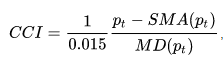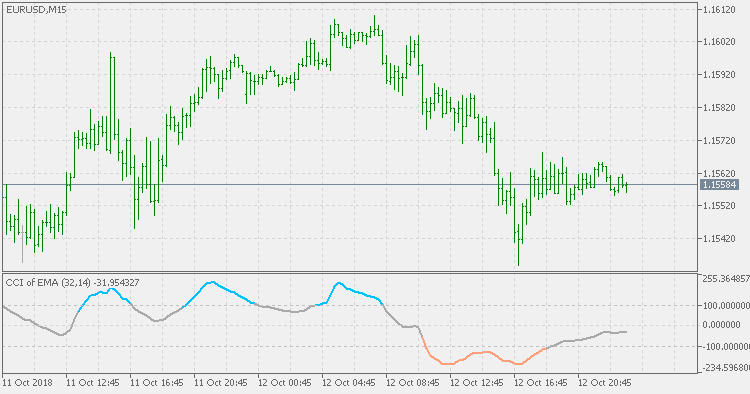Interesting script?
So post a link to it -
let others appraise it

You liked the script? Try it in the MetaTrader 5 terminal# CCI of average - indicator for MetaTrader 5

Views:
1908
Rating:
Published:
2018.10.13 19:26
Updated:
2019.01.29 14:50

Basics:

The commodity channel index (CCI) is an oscillator originally introduced by Donald Lambert in 1980.

CCI measures a security’s variation from the statistical mean.

The CCI is calculated as the difference between the typical price of a commodity and its simple moving average, divided by the mean absolute deviation of the typical price. The index is usually scaled by an inverse factor of 0.015 to provide more readable numbers:where pt is the typical price (high+low+close)/3, SMA is the simple moving average, and MD is the mean absolute deviation.

For scaling purposes, Lambert set the constant at 0.015 to ensure that approximately 70 to 80 percent of CCI values would fall between −100 and +100. The CCI fluctuates above and below zero. The percentage of CCI values that fall between +100 and −100 will depend on the number of periods used. A shorter CCI will be more volatile with a smaller percentage of values between +100 and −100. Conversely, the more periods used to calculate the CCI, the higher the percentage of values between +100 and −100.

This version:

Sometimes CCI is "nervous" - if you use it for signals based on levels crossing, it can produce a lot of false signals. One of the possible ways is to pre-filter the prices prior to being used in CCI calculation (that way adds significantly less lag then smoothing the already calculated CCI). This version does that : uses pre-filtered price for CCI calculation. You can use the usual set of averages types:

• simple moving average (SMA)
• exponential moving average (EMA)
• smoothed moving average (SMMA)
• linear weighted moving average (LWMA)

Usage:

Chose one of the 3 coloring modes (color change on slope, levels or zero cross) and then you can use the color change as signals.True Strength Index tradeTrue Strength IndexMomentum of averageDrunkards walk